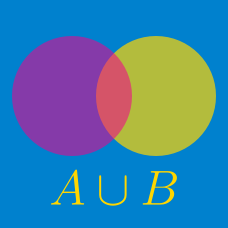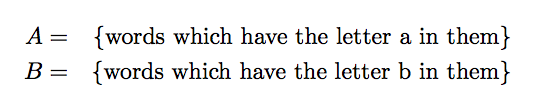Discrete Mathematics

# Sets - Composition of OperationsWhat is

$( A \backslash B ) \cup ( B \backslash A)?$

If $A=\{2,5,6,18,20\}, B=\{1,2,6,20,22\},$ then which of the following is equal to $$\left((A \setminus B) \cup (B \setminus A)\right) \cap A?$$

Details and assumptions

You may choose to read the summary page Set Notation.

Consider the ground set $U=\{x \mid x \mbox{ is a non-negative integer } \leq 9\}$ and let $$A$$ and $$B$$ be two subsets of $$U.$$ If $A^c \cap B^c=\{8, 9\},$ what is $$\lvert A \cup B \rvert?$$

The sets $A=\{12,14,16\}, B=\{13,15,17\}$ are subsets of a universal set $$U$$. If $$A \cup B=U,$$ then what is the sum of the elements of the set $\left((A \cap B) \cup (A^{C} \cap B)\right) \cup \left((A^{C} \cap B^{C}) \cup (A \cap B^{C})\right)?$

Details and assumptions

You may choose to read the summary page Set Notation.

Suppose $$A$$ and $$B$$ are the following subsets of a universal set $$U$$: $A=\{1,3,5,10,13,23\}, B=\{2,4,6,14\}.$ What is the number of elements of the set $A \setminus \left((A \setminus B) \cup \left(A \setminus B^{C}\right)\right)?$

Details and assumptions

You may choose to read the summary page Set Notation.

×

Problem Loading...

Note Loading...

Set Loading...